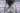# Recursion and DOM NodesEdit

This week I completed Eloquent JavaScript Chapter 14 (yay!). The second exercise in this chapter was pretty challenging but I'm proud of my solution.

The exercise was to create my own version of the `getElementByTagName` method. Before I get into my solution, let's go over some background information about the the Document Object Model (the "DOM").

## The DOM is a Tree

The DOM is basically a tree. The base node of the tree is `document`. Subsequent nodes are either "element" nodes or "text" nodes.

Element nodes can contain elements nodes, which can contain element nodes, and so on. Text nodes form "leaves" of the tree.

## Flattening a Node

To get all of the elements in a document/node that contain a given tag name, I needed to recursively search through each element in that node.

I broke the problem into two parts. First, I wanted to "flatten" a given node. Second, I wanted to filter out nodes that match a given element name (i.e., 'h1'). The second part is trivial so I'm leaving it out of this post.

Here is my solution for recursively flattening a dom node:

``````const flattenNode = (node) => {
const arr = Array.from(node.childNodes);
// reduce(callack = flatReducer, initialValue = [])
return arr.reduce(flatReducer, []);
}

// The first time the callback is called, accumulator and
// currentValue can be one of two values. If initialValue is
// provided in the call to reduce(), then accumulator will
// be equal to initialValue, and currentValue will be equal
// to the first value in the array.
const flatReducer = (accumulator, currentValue) => {
return accumulator.concat(
(currentValue.nodeType === Node.ELEMENT_NODE  && (!!currentValue.childNodes)) ?
[].concat(currentValue).concat(flattenNode(currentValue))
: currentValue
)
}``````

Thank you stack overflow for inspiration on how to use reduce to recursively flatten an array.

## Reduce

My solution makes use of the native JavaScript Array method `reduce`. As usual, mdn has great documentation on this method.

To summarize, the `reduce` method takes a callback function (a "reducer") and an initial value.

I defined the callback function ("flatReducer") separately to improve readability. The initial value is set to an empty array.

If the currentValue is an Element Node and it has child nodes, I recursively call the flattenNode function on that currentValue. Eventually everything gets concatenated and we get a flattened array of each element inside a dom node.

It still takes me a while to wrap my head around recursive functions, but it's so much fun to use.

I hope you've enjoyed this post!